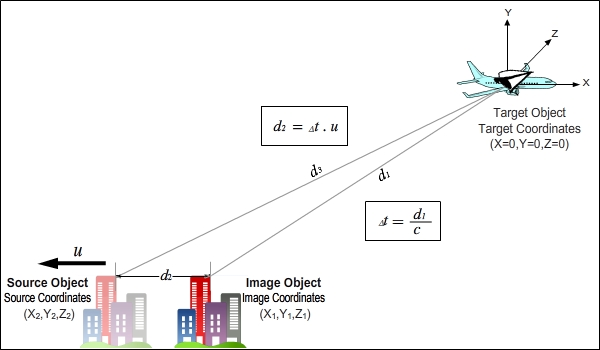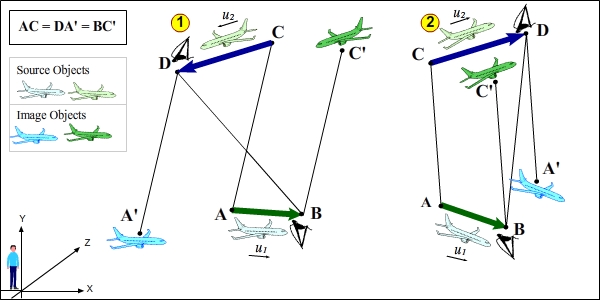18. IMAGE AND SOURCEImage and Source is, in a nutshell, a special and extensive topic that examines how the electromagnetic waves that carry images of objects are perceived by the receiver at the arrival target and what kinds of effects it causes.Image and Source has been known for a very long time, but I cannot say it is completely understood or known exactly because this topic is directly related to (c+v) (c-v) mathematics and the details of the topic can only be understood while thinking with this mathematics in mind. Since electromagnetic waves behave accordingly with the rules of (c+v) (c-v) mathematics, it is obvious that the topic Image and Source will be completely dependent upon the rules of (c+v) (c-v) mathematics.We can give a lot of examples for the signal receivers that perceive electromagnetic waves; our eyes as our biological organs, various devices (walkie-talkie, radar, TV antenna, radio, etc.) or any device that we use for observation (telescope, binoculars, etc.) are examples we can give. Additionally, all objects without exceptions are actually in the position of a signal receiver; for instance, we can consider even a stone as a signal receiver. Signal transmitters are, on the other hand, objects capable of sending electromagnetic waves and all objects without exceptions; they are everything that we see or cant see around us but that exists as objects. The fact that objects emit electromagnetic waves around them or receive electromagnetic waves coming to them puts them in a position of natural signal transmitters and receivers. At micro-level, signal receivers and transmitters are atoms that constitute objects.Three definitions are needed to cover the topic Image and Source. Source Object, Target Object, and Image Object.Source Object: The object that emits signals.  Target Object: The object that is the arrival target of the signal. Image Object: Is the image of Source Object.The relationship between three objects is as follows: Source Object sends the electromagnetic waves that form its own image to Target Object. Target Object, with the information the electromagnetic waves that reach it, sees the image of Source Object (Image Object).The best example that can be given for Source Objects and Image objects is, of course, the sky. When we look at the sky, we see Image Objects of stars. At that moment, Source Objects of the same stars are in utterly different places in the sky.  Source Objects are never visible under any conditions or in any cases. The things we see and perceive are Image Objects of Source Objects. This rule is valid for all devices that perceive signals and all objects inasmuch as for our eyes. Finding where the location of a Source Object is only possible by doing mathematical calculations.If Source Object and Target Object are in motion relative to each other, the coordinates where Source Object and Image Object differ for Target Object. The more the amount of speed of the movement and the distance between, the more this difference increase.The figure below represents the topic Image and Source.The course of events for the figure above is as follows: Source Object moves at u speed in the direction of the arrow.  When Source Object is at (x1,y1,z1) point (the location where the Image Object is in the figure), the electromagnetic waves forming it (the signal) set out.  When the signal reaches Target Object (the observer) that is in (x=0, y=0, z=0), the observer sees Image Object (the image of the plane). The place where Image Object is seen is (x1,y1,z1) point that is the coordinate of the signal emission. The signal reaches the observer in Δt= d1/c duration and Source Object will travel d2=Δt.u distance in Δt duration. Therefore, at the moment the observer sees the plane, the coordinates of Source Object become (x2,y2,z2). Attention should be paid to the fact that d1,d2 and d3 lines form a Doppler Triangle.  Although the topic Image and Source seems simple, when we go into the details of the topic a bit, we easily see that it is not a simple topic at all. Id like to show this to you with a question.Question: Lets assume that there is another observer looking to the city through the window of the plane in the figure. At the moment the observer on the ground sees the image of the plane at (x1,y1,z1) coordinates, where does the observer on the plane see Image of the City?Things got difficult all of a sudden, didnt they? What does at the same time mean? How do the signals that belong to the city go to the plane? At which angle do the signals reach the plane? How can we find Image of the City? Does the d3 length have a special meaning? Questions like these come to the fore on their own.Principles, principles, principles I think how important are the principles and that we should never lose them from our sight will be understood here very well. Lets see how easily we can answer the questions above when we use the principles for them. For this, lets assume that the reference system of the plane is motionless.The figure above is prepared relative to the reference system of the plane. Lets write the course of events in order just in the first example. Source Object (the city) moves at u speed in the direction of the arrow.  When Source Object is at (x1,y1,z1) point, the electromagnetic waves forming its image set out.  When the signal reaches Target Object, namely the observer, that is in (x=0, y=0, z=0), the observer sees Image Object (the image of the city). The coordinates where Image Object is seen is (x1,y1,z1) point which is the coordinate of the signal emission. The signal reaches the observer on the plane in Δt= d1/c duration and Source Object will travel d2=Δt.u distance in Δt duration. Therefore, at the moment the observer sees the city, the coordinates of Source Object become (x2,y2,z2).  There are few important details Id like to direct your attention to. The signal comes to Target Object itself by following the d1 line. Therefore, the one that determines at which angle the signal arrives the target is d1 line. d3 is the direction of movement relative to the reference system of Source Object. d3 has no effect on the arrival angle of the signal at the target.  Id like to draw your attention to the fact that the triangle whose sides are set by d1, d2 and d3 lines is Doppler Triangle that we saw in the part where we covered Doppler Shift. The multiplication of the speed of the signal emitted relative to the reference system of Source Object and the travel time is equal to d3 distance.  d3 = Δt . c' = Δt . (c+v) (As the frames are moving away from each other here, the speed of the outgoing signal is (c+v).) From here on in, our job is easy. Now that we know the location of Image Object of the city relative to the planes reference system, if we transfer this location to the first figure, we will have given an answer to the question Where does the observer on the plane see the city?In the figure above, the coordinates where the observer on the plane sees the image of the city relative to the reference system of the observer on the ground are represented. The places where both the observers are inside Source Objects that represent the real objects. Doppler Triangles have been formed not only for the observer on the plane but also the observer on the ground (the triangles formed by d1, d2, and d3 lines). While the observer on the ground sees the image of the plane, the observer on the plane sees the image of the city.Where Image Objects are seen can be easily found with the help of Doppler Triangles or Doppler Quadrangles. In the visual on the left in the figure above, as the planes are coming towards each other, Doppler Quadrangle is folded on its two sides. The quadrangle in the visual on the right is seen more clearly because the movements are in the same direction. The direction and length of AC line that connects point A and C that signals emit mutually serve as a reference for the locations of image objects. The parallel lines to AC lines that have the same length and that are drawn from point B and D, which are the destinations of the signals, gives the locations of Image Objects. Lets pay attention to the fact that AC line in the figure is the travel direction of the incoming signal from both the reference systems. For this reason, the lines that are drawn parallel to AC line determine the locations of image objects.As can be seen in the figure above, if we connect point A'BC'D and BA'DC', parallelograms are formed. When the coordinates of Source Object and Image Object are connected, a parallelogram is formed at all times. Always the same types of objects are present at the ends of diagonals of the parallelogram. Source Object matches with Source Object and Image Object matches with Image Object. If the locations of three objects are known, the location of the fourth object can be easily found by making use of parallelogram method.In the figure on the left side above, we will find where the observer on the plane sees the other plane by using the reference system of the observer on the ground as a base.Doppler Quadrangle (The left visual in the figure) The coordinates are relative to the reference system of the man on the ground. Point A and C are the beginning of the event.  Point A is the point at which the signal is emitted. Point B and D are the locations of the planes at the moment of the signal arrival.  DA' line that is parallel to AC line and that has the same length gives the location of Image Object.  The observer on the plane at point D sees the image of the plane at point A'. In the figure on the right above, we will find where the observer on the plane sees the city by using its own reference system as a base.Doppler Triangle (The right visual in the figure) The coordinates are relative to the reference system of the observer on the plane at the top. The observer assumes that its own reference system is motionless and that the city is in motion. Point A and B are the beginning of the event.  Point A is the point of signal emission. Point A and C are the locations of the city and the plane at the time of signal arrival. CA' line that is parallel to AB line and that has the same length gives the location of Image Object.  The observer at point C sees the image of the plane at point A'.The figures had to be drawn with extreme exaggeration. If we consider that the speed of light is 300.000 km/sec, the distances shown in colored arrows in the figure above are very short in reality.